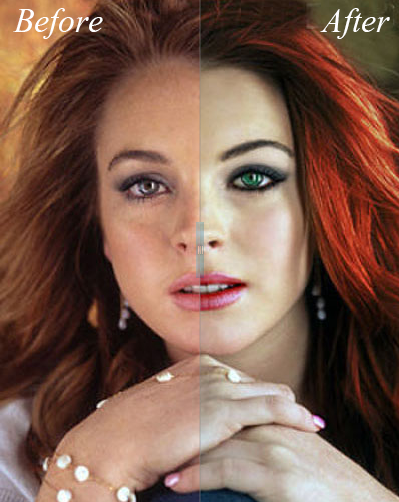Mode Gelap
Utilities

Before/After Photo Effect with jQuery Blogger Photo Editing

If you have a design or makeup blog, or if you are using before and after image comparison, this script will most likely be useful for you. In this tutorial, you will see how to add the Before/After plugin, a script that works with jQuery in order to display two pictures dynamically for comparing them at the same time.

To see how it works, click on the demo link from below and drag horizontally the small bar to see both images that are being compared:Implementing this script is really easy. We just need jQuery, the Before/After script, and the two images to compare.

How to add Before/After Effect on Blogger Images

Step 1. Go to "Template" and click on the "Edit HTML" button. Once the template HTMK editor opens up, click anywhere inside the code area and press the CTRL + F keys to search for the following tag - hit Enter to find it:Step 2. Right before </head> paste the following scripts:
<script src='http://code.jquery.com/ui/1.10.3/jquery-ui.js' type='text/javascript'/>
<script type='text/javascript'>
//<![CDATA[
(function(a){a.fn.extend({beforeAfter:function(b){var d={animateIntro:false,introDelay:1000,introDuration:1000,showFullLinks:true,imagePath:"/js/beforeAfter/"};var b=a.extend(d,b);var c=Math.round(Math.random()*100000000);return this.each(function(){var e=b;var h=a(this);var n=a("img:first",h).width();var p=a("img:first",h).height();a(h).width(n).height(p).css({overflow:"hidden",position:"relative",padding:"0"});var m=a("img:first",h).attr("src");var j=a("img:last",h).attr("src");a("img:first",h).attr("id","beforeimage"+c);a("img:last",h).attr("id","afterimage"+c);a("img",h).remove();a("div",h).css("float","left");a(h).prepend('<div id="dragwrapper'+c+'"><div id="drag'+c+'"><img width="8" height="56" alt="handle" src="http://4.bp.blogspot.com/-BSOVMuAgJgU/Umz0-AsGtyI/AAAAAAAAEdI/dXcdcClGu6w/s1600/handle.png" title="Drag to the left or right to see the Before/After" id="handle'+c+'" /></div></div>');a("#dragwrapper"+c).css({position:"absolute",padding:"0",left:(n/2)-(a("#handle"+c).width()/2)+"px","z-index":"20"}).width(a("#handle"+c).width()).height(p);a("#dragwrapper"+c).css({opacity:0.25});a("div:eq(2)",h).height(p).width(n/2).css({"background-image":"url("+m+")",position:"absolute",overflow:"hidden",left:"0px","z-index":"10"});a("div:eq(3)",h).height(p).width(n).css({"background-image":"url("+j+")",position:"absolute",overflow:"hidden",right:"0px"});a("#drag"+c).width(2).height(p).css({background:"#888",position:"absolute",left:"3px"});a("#beforeimage"+c).css({position:"absolute",top:"0px",left:"0px"});a("#afterimage"+c).css({position:"absolute",top:"0px",right:"0px"});a("#handle"+c).css({"z-index":"100",position:"relative",cursor:"pointer",top:(p/2)-(a("#handle"+c).height()/2)+"px",left:"-3px"});a(h).append('<img src="http://4.bp.blogspot.com/-nztJPbkautM/Umz1AFOuJZI/AAAAAAAAEdU/-_LYBKwoKDM/s1600/b_a-lt-small.png" width="7" height="15" id="lt-arrow'+c+'"><img src="http://4.bp.blogspot.com/-iWaLPmPPAU8/Umz0_PLDCtI/AAAAAAAAEdM/Zam6QWGabVM/s1600/b_a-rt-small.png" width="7" height="15" id="rt-arrow'+c+'">');if(e.showFullLinks){a(h).after('<div class="balinks" id="links'+c+'" style="position:relative"><span class="bflinks"><a id="showleft'+c+'" href="javascript:void(0)">Show only before</a></span><span class="bflinks"><a id="showright'+c+'" href="javascript:void(0)">Show only after</a></span></div>');a("#links"+c).width(n);a("#showleft"+c).css({position:"relative",left:"0px"}).click(function(){a("div:eq(2)",h).animate({width:n},200);a("#dragwrapper"+c).animate({left:n-a("#dragwrapper"+c).width()+"px"},200)});a("#showright"+c).css({position:"absolute",right:"0px"}).click(function(){a("div:eq(2)",h).animate({width:0},200);a("#dragwrapper"+c).animate({left:"0px"},200)})}var g=a("#dragwrapper"+c).offset();var k=g.left;var i=a("div:eq(2)",h).width();var f=a("div:eq(3)",h).width();a("#dragwrapper"+c).draggable({handle:a("#handle"+c),containment:h,axis:"x",drag:function(q,o){var s=a(this).offset();var r=s.left-k;a("div:eq(2)",h).width(i+r);a("#lt-arrow"+c).stop().animate({opacity:0},0);a("#rt-arrow"+c).stop().animate({opacity:0},0)}});if(e.animateIntro){a("div:eq(2)",h).width(n);a("#dragwrapper"+c).css("left",n-(a("#dragwrapper"+c).width()/2)+"px");setTimeout(function(){a("#dragwrapper"+c).css({opacity:1}).animate({left:(n/2)-(a("#dragwrapper"+c).width()/2)+"px"},e.introDuration,function(){a("#dragwrapper"+c).animate({opacity:0.25},1000)});a("div:eq(2)",h).width(n).animate({width:n/2+"px"},e.introDuration,function(){l()})},e.introDelay)}else{l()}function l(){a(h).hover(function(){a("#lt-arrow"+c).stop().css({"z-index":"20",position:"absolute",top:p/2-a("#lt-arrow"+c).height()/2+"px",left:parseInt(a("#dragwrapper"+c).css("left"))-10+"px"}).animate({opacity:1,left:parseInt(a("#lt-arrow"+c).css("left"))-6+"px"},200);a("#rt-arrow"+c).stop().css({position:"absolute",top:p/2-a("#lt-arrow"+c).height()/2+"px",left:parseInt(a("#dragwrapper"+c).css("left"))+10+"px"}).animate({opacity:1,left:parseInt(a("#rt-arrow"+c).css("left"))+6+"px"},200);a("#dragwrapper"+c).animate({opacity:1},200)},function(){a("#lt-arrow"+c).animate({opacity:0,left:parseInt(a("#lt-arrow"+c).css("left"))-6+"px"},350);a("#rt-arrow"+c).animate({opacity:0,left:parseInt(a("#rt-arrow"+c).css("left"))+6+"px"},350);a("#dragwrapper"+c).animate({opacity:0.25},350)});a(h).click(function(q){var r=q.pageX-this.offsetLeft;var o=n-r;a("#dragwrapper"+c).stop().animate({left:r-(a("#dragwrapper"+c).width()/2)+"px"},600);a("div:eq(2)",h).stop().animate({width:r+"px"},600);a("#lt-arrow"+c).stop().animate({opacity:0},50);a("#rt-arrow"+c).stop().animate({opacity:0},50)});a(h).one("mousemove",function(){a("#dragwrapper"+c).stop().animate({opacity:1},500)})}})}})})(jQuery);
//]]>
</script>
<script type='text/javascript'>
\$(function(){
});
</script>
Step 3. Click the "Save template" button to save your changes.

Note: if the above script doesn't work for you, add it above the </body> tag. Also, please see if you have added jQuery in your template (if you did so, then remove the line in green).

Step 3. To add it as a gadget - go to "Layout" and click on the "Add a gadget" link, then choose "HTML/Javascript" option. Paste this code inside the empty box:
<div id='beforeafter1'>
<div><img alt='before' src='URL of the first imagewidth='500' height='291'/></div>
<div><img alt='after' src='URL of the second imagewidth='500' height='291'/></div>
</div>
Add the URL of the first image that is the "Before" image, and the URL of the second image that should be the "After" image. Also, don't forget to specify the width and height of each picture, so that they work on all browsers (see the part in orange where you need to add the height and width of your images).

If you want to add more images, you will need to change the ID of the container. In my example, the container is called beforeafter1, so you will need to change it to beforeafter2 and so on, although, you can choose any name.

Then, add another line, like the blue one from below, in the first code that you added in step 2:
<script type='text/javascript'>
\$(function(){
});
</script>
You can add as many before/after images as you want, as long as all containers have a different ID and the corresponding lines are added in the script.

Below each image /photo container are the "Show only Before" and "Show only after" links that once clicked, will display the "before" or "after" picture. If you want to hide these links, then just change "true" to "false" in the first code (step 2) and they will not be displayed anymore.

Note: if you see white space around the drag icons, then search for this code in your template:
<Group description="Images" selector=".main-inner">
<Variable name="image.background.color" description="Background Color" type="color" default="#ffffff" value="#ffffff"/>
And change the #ffffff value to transparent."Dengan berbicara di belakang, berarti kau cukup menghargai keberadaanku untuk tidak bertingkah di depan mukaku."